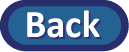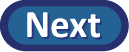## Step 1. Ask a research question.

For this study, the authors thought the average at your school would be below the recommended 8 hours. So our research question is: is there convincing evidence that students at your school tend to sleep less than 8 hours on a typical night?

(a) Based on our research question:

• What is the population of interest?
• What is the parameter?
• What symbol do we use for this parameter?

## Step 2. Design a study and collect data.

(b) Describe the characteristics of the sample being used in this study.

(c) Define the variable that was measured on each observational unit.

(d) To investigate whether the mean amount of sleep last night for the population of all students at your school was less than 8 hours:

• What is the null hypothesis?
• What is the alternative hypothesis?